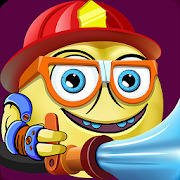# Math rescue: Mental Math Practice Cool Math Games

Makkajai: Math Games for 1st, 2nd, 3rd, 4th grade | 10,000+ Installs

3.725 ratings

Math rescue: Mental Math Practice is consisted of 3rd-grade math and 4th-grade math games. It is a cool math playground where kids can solve math problems and improve their math skills in addition, subtraction, multiplication, and other math facts. Fast math learning with this kids math app is fun!

Singapore math inspired, these cool math games combine fun gameplay with strong pedagogy to help your child practice rounding and estimation. Fast math learning can be achieved using these techniques, and with enough practice, kids will be able to do approximations in their head really quickly.

Mental math helps children to solve mathematical problems without using a calculator or paper and pencil.

3rd-grade math and 4th-grade math are about building upon your child’s foundational knowledge of addition, multiplication, subtraction, and division. Keep the math practice interesting and your child engaged with our app! Let it play with fast math for kids!

Math practice is essential to learn any new concept, and these cool math games are no different – once the child is introduced to the mental math techniques of rounding, the game drills them through a variety of math problems; all in a fun and effective game format. Exciting gameplay with great sound and art will keep kids engaged for a long time.

Main features of these mental math games:
✔️ Kids math playground - Kids will have math practice in a fun and entertaining way, and they will love to spend time with our cool math games and to do the math learning.
✔️ Helps your child to achieve fast math techniques so they can do addition, division, subtraction, multiplication and other operations in their head quickly. Mental math helps kids to solve math problems without using a calculator
✔️ With this app kids are learning math skills such as: rounding to nearest 10s and 100s of two and three digit numbers, estimating sum of two 2-digits and 3-digit numbers.
✔️ Beyond those skills, kids learn how to round up numbers, and they will understand more basic math concepts such as the place value of a digit in a number. The app reinforces these math concepts with self-paced practice anytime, anywhere.
✔️ The game is designed for 3rd-grade math and 4th-grade math level, but it is also suitable for 5th-grade math level.
✔️ This mental math learning game is aligned to common core math and Singapore math

Estimation, especially fast estimation of addition of multiple numbers, is an essential skill in the daily life application of Math. Use our kids math game to form a solid foundation for your child’s math skills.

Math facts and skills covered:
⭐ Rounding to nearest 10, and to nearest 100
⭐ Rounding up and down
⭐ Rounding 2 digit numbers to nearest 10, and 3 digit numbers to nearest 10
⭐ Rounding numbers with 5 in the units place
⭐ Rounding 3 digit numbers to the nearest 100
⭐ Estimating addition of two 1-digit numbers, two 2-digit numbers, and two 3-digit numbers
⭐ Estimating addition of one 2-digit and one single digit number
⭐ Estimating addition of one 2-digit and one 3-digit number

The skills covered, correspond to the following common core curriculum standards:
✔️ 3.NBT.A.1 - 3rd-grade math - Use place value understanding to round whole numbers to the nearest 10 or 100.
✔️ 4.NBT.A.3 - 4th-grade math - Use place value understanding to round multi-digit whole numbers to any place.

Give your child a reason to enjoy in the mental math practice and it will master fast math techniques quicker than you think!